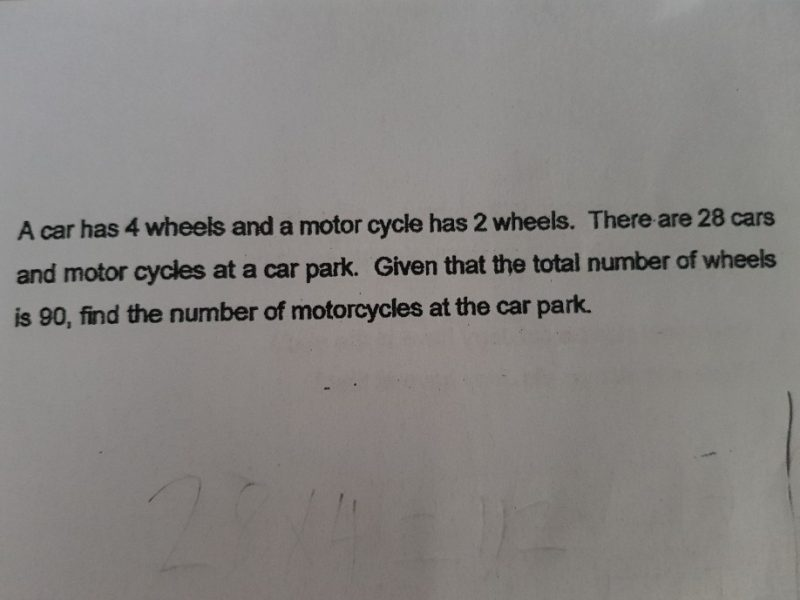QuestionHi, can someone help with this question. Thx

If all 28  vehicles were cars, total wheels = 28 x 4 = 112 wheels.

Only 90 wheels are there, so 112 – 90 = 22 wheels.

Number of motor cycles = 22/2 = 11

Cars = 28 – 11 = 17.

Verify

17 x 4 = 68

11 x 2 = 22

Total wheels = 68 + 22 = 90

=============================================================

www.orckids.biz, ph:87508449

1 Reply 0 Likes

You make it so simple to understand. Thanks so much.

0 Replies 0 Likes